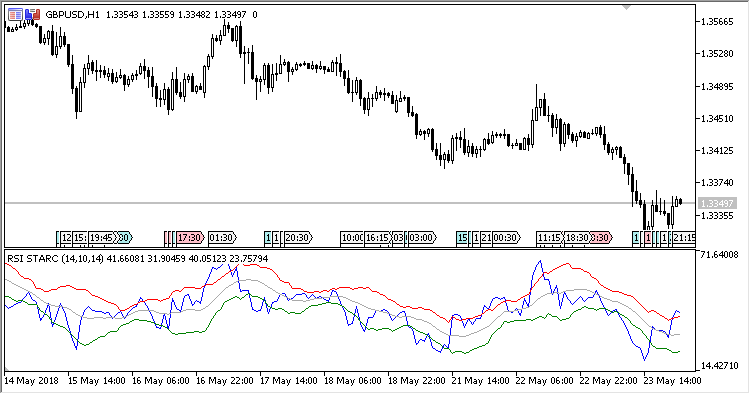# RSI_STARC – indicator for MetaTrader 5

The indicator represents a combination of RSI and STARC (Stoller Average Range Channels), which is similar to indicator Bollinger Bands in its behavior: Its bands get narrower on stable markets and wider on volatile markets.

However, STARC is not based on close prices and it does not consider standard square deviation. It is calculated using average true range (ATR), providing more detailed information on market volatility.

The indicator has five input parameters:

• RSI period – RSI calculation period;
• ATR period – ATR period for calculating STARC;
• Smoothing – smoothing the central line of the STARC channel;
• Top line multiplier – multiplier of the channel top line (range upwards from the mean line);
• Bottom line multiplier – multiplier of the channel bottom line (range downwards from the mean line).
Related Posts

Calculations:

`RSI = Relative Strength Index(Close price, RSI period)`
```MA = MA(RSI, Smoothing)
Top = MA + Top line multiplier * ATR
Bottom = MA -Bottom line multiplier * ATR
```

where:

`ATR = MA(TR, ATR period)TR[i] = Abs(RSI[i] - RSI[i-1])`